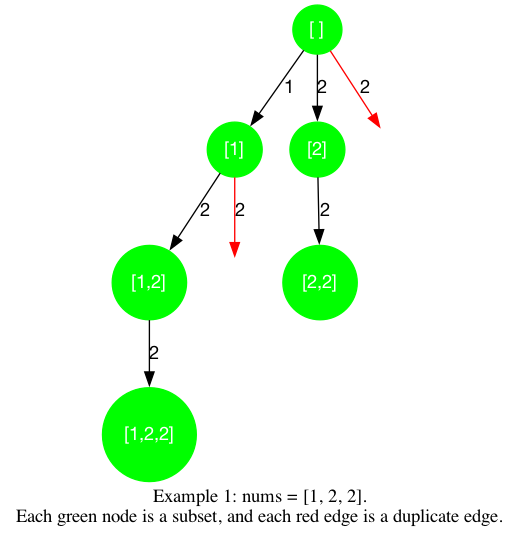# LeetCode Subsets II Solution

Given an integer array `nums` that may contain duplicates, return all possible subsets (the power set).

The solution set must not contain duplicate subsets. Return the solution in any order.

Example 1:

Input: `nums = [1,2,2]`

Output: `[[],,[1,2],[1,2,2],,[2,2]]`

Example 2:

Input: `nums = ` Output: `[[],]`

Constraints:

• `1 <= nums.length <= 10`
• `-10 <= nums[i] <= 10`

## Solution

This question is an advanced version of Subsets, the only difference is that the input may contain duplicate elements. So we can use the same approach as in Subsets to find the subsets of `nums`. However, we still need to deduplicate the repeated elements. In Deduplication we learned that we can deduplicate by sorting the candidates and avoiding using a duplicate candidate that we have not used previously, we will do the same here.

We wish to fill in the logics for backtracking1 template.

• `is_leaf`: all the paths is a subset of `nums`
• `get_edges`: the potential edges are the numbers from `nums[start_index:]` or an empty edge that concludes the subset
• `is_valid`: check whether the candidate `nums[i]` is the first appearance of that element in the current function call, that is, `i > start_index and nums[i] == nums[i-1]` is `true` when `nums[i]` is a duplicate, and `false` otherwise.

Since at every node, a special "edge" is to "close" the subset, we can add a copy of `path` to `ans` regardless of the value of other states. Then, when we `get_edges` we can consider only the numbers from `nums[start_index:]`, as we have visited `nums[0:start_index]`.

#### Implementation

``````1def subsetsWithDup(self, nums: List[int]) -> List[List[int]]:
2    def dfs(start_index, path):
3        ans.append(path[:]) # add a copy of the path to the result
4        for i in range(start_index, len(nums)):
5            # prune if needed
6            if i > start_index and nums[i] == nums[i-1]:    # avoid duplicates
7                continue
8            path.append(nums[i])
9            dfs(i + 1, path)
10            path.pop()
11
12    ans = []
13    nums.sort()
14    dfs(0, [])
15    return ans``````